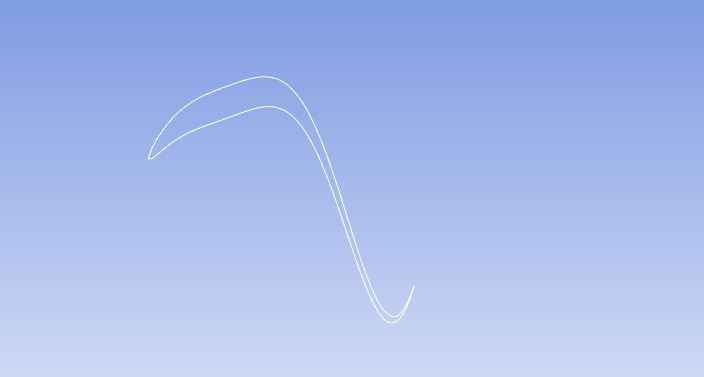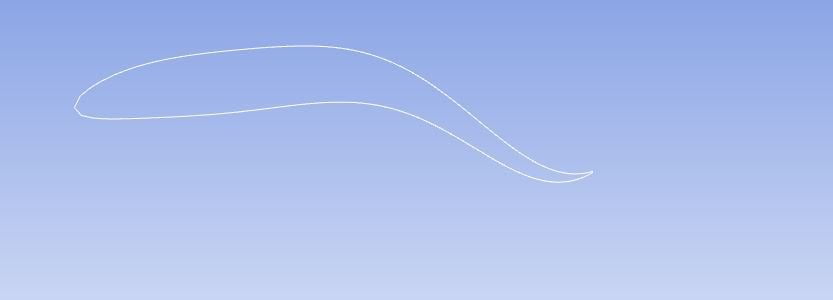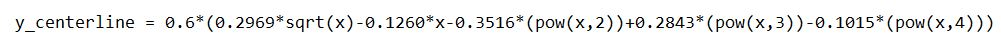## Fluids

Topics relate to Fluent, CFX, Turbogrid and more

•MohamedAyman1
Subscriber

Hello!!

I hope this message finds you all well. I am working on a 2D case of airfoil shape (NACA0012) and trying to make the undulation motion of the fish using GRID_MOTION macro. I have created the UDF and compile it successfully however, Instead of moving the Walls of the airfoil, the centerline is moving with the expected motion. I have attached two photos; one for the airfoil and the other is for the Centerline movement. How can I solve this problem to give the motion to the airfoil wall not the centerline?.

•YasserSelima
Subscriber
Why are you using GRID_MOTION? grid_motion is mainly designed if the shape of the body changes ... inflation, shrinkage, bending .. etc. If the shape of the airfoil remains constant, and it only rotates, and translates .. you don't need to Use Grid_motion.nnI recommend using DEFINE_CG_MOTION ... this would shorten your code.n
•MohamedAyman1
Subscriber
Dear Yasser,nThanks for replying. Actually the airfoil body is changing and cannot be treated as a rigid body that only rotates and translates which is knowing as flapping motion (pitching + plunging). DEFINE_CG_MOTION is the perfect choice for that case as you said but, in my case, the airfoil shape is changing with time to generate the undulation motion a s illustrated in figure 2. My problem is that the centerline is moving with the motion instead of the airfoil's wall.nn
•YasserSelima
Subscriber
In this case, you are right ...GRID_MOTION is the right choice. nSo, why is the centerline defined in Fluent? Are you defining the airfoil body as a solid zone?
•MohamedAyman1
Subscriber
Dear Yasser,nThe centerline is not defined in FLUENT and the airfoil's wall is defined in named selection. I really don't know why the airfoil itself does NOT undulate. The airfoil is subtracted from the fluid domain so it is all fluid. ANY HELP?!!nn
•YasserSelima
Subscriber
I am not sure why is this happening .. seems weird ... one more question, how did you recognise that the centerline is moving?.For debugging,I would suggest you simplify the motion and see if the problem still occur. Like give it a translation motion ... Also you can print the NODE_Y before and after the update and see if the values change like what you want or not. nI might be able to help you debugging if you don't mind posting the UDFnn
•MohamedAyman1
Subscriber
Dear Yasser,nThanks for offering help. Actually, I tried the rotating motion of the airfoil (Pitching mechanism) by  DEFINE_CG_MOTION and the motion was as expected but when I try the undulation motion by DEFINE GRID_MOTION the airfoil two lines merges to one line which seems to be the centerline. I didn't understand what do you mean f print NODE_Y before the update. Below is the UDF:n#include udf.hn#include dynamesh_tools.hn#include math.hn#define U 40.05n#define L 1.00n#define a0 0.02n#define a1 0.08n#define a2 0.16nnDEFINE_GRID_MOTION(Fish_movment, domain, dt, time, dtime)n{nThread *tf = DT_THREAD(dt); /* pointer to a thread*/nface_t f;nint n;nNode *node_p;nreal A, x, y, wave, sign;nreal St = 0.05;n/* set deforming flags */nSET_DEFORMING_THREAD_FLAG(THREAD_T0(tf));  /* means that the nodes adjacent to the moving wall will be defrormed to avoid skewness*/nbegin_f_loop(f, tf)n{nf_node_loop(f, tf, n)n{nnode_p = F_NODE(f, tf, n);n/* Update the current node only is not been visited before*/nnif (NODE_POS_NEED_UPDATE(node_p))n{n/*Set flag to indicate that the node position has been updated*/nNODE_POS_UPDATED(node_p);nx = NODE_X(node_p);nA = a0 - a1*x + a2*x*x;nwave = 2 * M_PI / L;nsign = x - St * U * time;ny = A * sin(wave*sign);nNODE_Y(node_p) = y;n}n}n}nend_f_loop(f, tf);n}nnI really appreciate your help.Arrayn
•YasserSelima
Subscriber
nTry this and see if you have a difference or not. Change the NODE_Y(node_p) = y; to NODE_Y(node_p) += y;nnLet me known
•MohamedAyman1
Subscriber
Dear Yasser,nReally thanks for your help. The code works and the airfoil's wall is moving instead of only one line. But, there is something strange happening, the airfoil is stretched in a strange shape as illustrated in Figure 1. But when I use a time step of 0.05 (For viewing the motion only) the motion looks good as illustrated in figure 2. When coming to setup and solving, I expect to decrease the time step for solver stability and validation of the results also. To conclude what I said:n1) The motion below 0.05 is not correct and the airfoil is stretched.n2) The motion is occurring on one side only.n3) Is there is something wrong with the UDF?nAgain thank you for your help.nn
•YasserSelima
Subscriber
Hello,nYou are welcome.n1) I am not sure why is this happening. The amplitude is not function of time. So this is really odd. Can you review all the equations , specially the amplitude A ... and try view motion with small time step and see if same thing is repeated or notn2) The second image you posted shows clearly that motion is happening on the two sides. Start from loading the original geometry ... nadd this below Node_y(node_p) linen#if RP_HOSTnMessage( x = %f , y = %f
, x, y);n#endifnRun two time steps only ... this should print the values of x and y of all nodes and see if they are updated right or not. Depending on your mesh size, expect to have many lines printed ... so, run it for two time steps only.nn3) I don't think there is something wrong
•MohamedAyman1
Subscriber
Dear Yasser,nSorry or the late reply. Again, I really appreciate your effort in helping me. Here are what I done:n1) I checked the amplitude equation again and there is nothing wrong in the equation.n2) I tried to change the strouhal number to see if the stretching foil is occurring or not but the same thing happened.n3) The lower the time step, the more the stretching of the foil.n4) The motion is occurring on only one side and then moves to equilibrium position and moves to the same side not the other one.n5) I didn't understand the benefit of:n#if RP_HOSTnMessage( x = %f , y = %f
, x, y);n#endifn6) Is it possible to share the Case file with you to understand what I mean?. Just sent me your mail.nAgain thank you for you helpArrayn
•YasserSelima
Subscriber
Hello,nNo need for sending the case. I think I did understood the issue. Here is my suggested solution .. This solution uses y_centerline as the position of the centerline.. I am assuming you know it as a function of xnIncrease the number of User Defined Node Memory locations to 1 before compiling the code.nBefore starting the simulation you need to save in the N_UDMI how far are they from the centerline ... Could be done by DEFINE_EXCUTE_ON_DEMANDnIn your GRID_MOTION function, you will have NODE_Y(node_p) = y + N_UDMI(f,tf,0);nnHere is an example of the define_on_demandn nDEFINE_EXECUTE_ON_DEMAND(init_value)n{n n Domain *d; n Thread *tf;n face_t f;n Node *node_p;n int n;n real x, y_centerline;n begin_f_loop(f, tf)n d = Get_Domain(1); n? tf = Lookup_Thread(d,zone_ID);n {n f_node_loop(f, tf, n)n {n node_p = F_NODE(f, tf, n);nt x = NODE_X(node_p);n y_centerline = ... ; /* calculate centerline position as f(x) */ n N_UDMI(node_p, 0) = NODE_Y(node_p) - y_centerline; n }n }n}nnIn the above, you need to addnzone_ID you can get this from the boundary conditions ... ny_centerline equation in terms of x .. if it is constant, just put the value.nnAnd definitely check syntax errors and typos ...nnLet me know if it works. Good Luck!n
•YasserSelima
Subscriber
Don't forget to run the function before the simulationn
•YasserSelima
Subscriber
did it work?n
•MohamedAyman1
Subscriber
Dear Yasser,nI hope thismessage finds you well. Actually the DEFINE_EXCUTE _ON_DEMAND macro cannot be interpreted nor compiled successfully. I followed your instructions well and nput the zone ID and the equation of the airfoil itself to define the distance from the centerline which is attached in the next figure to check it:nBut the DEFINE_EXCUTE _ON_DEMAND cannot be into the solver successfully. I checked the syntax and added #include udf.h and #include math.h before your code and still no results. I removed the EXCUTE word as said in the UDF manual but no result. Cannot know the exact problem!!!!!. Any ideas?nAgain, thanks for your help.nMohamed n
•YasserSelima
Subscriber
DEFINE_ON_DEMAND
•YasserSelima
Subscriber
what is the error message?ntry it like thisnn#include udf.hnnDEFINE_ON_DEMAND(init_value)n{n   n   Domain *d; n   Thread *tf;n   face_t f;n   Node *node_p;n   int n;n   real x, y_centerline;n d = Get_Domain(1);     n﻿   tf = Lookup_Thread(d,zone_ID);   begin_f_loop(f, tf)n      {n       f_node_loop(f, tf, n)n       {n           node_p = F_NODE(f, tf, n);nt   x = NODE_X(node_p);n           y_centerline = ... ;               /* calculate centerline position as f(x) */ n           N_UDMI(node_p, 0) = NODE_Y(node_p) - y_centerline; n      }n  }nnn
•MohamedAyman1
Subscriber
Dear Yasser,nThanks for the fast reply. The UDF cannot be compiled nor interpreted successfully. Attached is the UDF for you to check it:n#include udf.hnnDEFINE_ON_DEMAND(init_value)n{n  n  Domain *d; n  Thread *tf;n  face_t f;n  Node *node_p;n  int n;n  real x, y_centerline;n  d = Get_Domain(1);   n  tf = Lookup_Thread(d,2);  begin_f_loop(f, tf)n   {n    f_node_loop(f, tf, n)n    {n      node_p = F_NODE(f, tf, n);nt  x = NODE_X(node_p); n      y_centerline = 0.6*(0.2969*sqrt(x)-0.1260*x-0.3516*(pow(x,2))+0.2843*(pow(x,3))-0.1015*(pow(x,4))) ;        n      N_UDMI(node_p, 0) = NODE_Y(node_p) - y_centerline ; n   }n }n}nnthe error message is attached in the next figure when I interpret the UDF:n